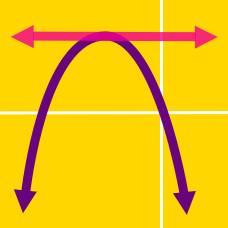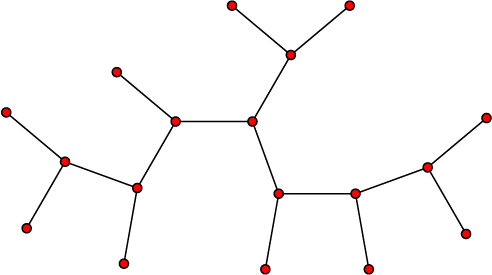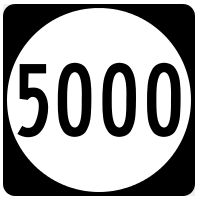Algebra

# Completing the Square: Level 4 Challenges

The numbers $a$ and $b$ are positive integers both greater than 5. The function $f(x) = \cos ^2 x - a \sin x + b$ has a maximum of $34$ and a minimum of $12$. What is the value of the product $a \times b$?

The Queen plans to build a number of new cities in the wilderness, connected by a network of roads. She expects the annual tax revenues from each city to be numerically equal to the square of the population. Road maintenance will be expensive, though. The annual cost for each road is expected to be numerically equal to the product of the populations of the two cities that road connects. To keep the costs for road maintenance down, the Queen decrees that there should be only one way to get from any city to any other, and not more than three roads should connect to any given city (the attached design would be acceptable, for example).

Unfortunately, Parliament is responsible for deciding which roads will be built (subject to the two Queen's decrees) and how many people live in each city, but has no concern for attempting to stay financially solvent (meaning tax revenue $\geq$ road maintenance). Regardless of what Parliament decides, what is the largest number of cities that the Queen can build without risking to lose money?

Inspiration$P(x)=(x^2+5000x-1)^2+(2x+5000)^2$

Let $P(x)$ be a polynomial, then find the sum of all the real solutions to the equation $P(x)=\text{min}(P(x)).$

###### Image Credit: Wikimedia Five Thousand

If $x, y, z \in\mathbb{R}$

$x^2+11z=-40.25$ $y^2-9x=-28.25$ $z^2+7y=5.75$

Then the value of $|x|+|y|+|z|$ is

Note

You can find more such problems here

How many values of $\lambda$ lie between $[-1000\pi,1000\pi]$ for which the polynomial $x^4-2^{\tan\lambda}.x^2+(\cos\lambda+\cos2\lambda).x+2^{\tan\lambda-2}$ is the square of the quadratic trinomial with respect to $x$ ?

×